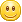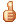# poj3682:数学期望，O(1)做法附推导过程

2016年5月10日 2.61k 次阅读 0 人点赞

King Arthur's Birthday Celebration
 Time Limit: 1000MS Memory Limit: 65536K Total Submissions: 2921 Accepted: 926

Description

King Arthur is an narcissist who intends to spare no coins to celebrate his coming K-th birthday. The luxurious celebration will start on his birthday and King Arthur decides to let fate tell when to stop it. Every day he will toss a coin which has probability p that it comes up heads and 1-p up tails. The celebration will be on going until the coin has come up heads for K times. Moreover, the king also decides to spend 1 thousand coins on the first day's celebration, 3 thousand coins on the second day's, 5 thousand coins on the third day's ... The cost of next day will always be 2 thousand coins more than the previous one's. Can you tell the minister how many days the celebration is expected to last and how many coins the celebration is expected to cost?

Input

The input consists of several test cases.
For every case, there is a line with an integer K ( 0 < K ≤ 1000 ) and a real number p (0.1 ≤ p ≤ 1).
Input ends with a single zero.

Output

For each case, print two number -- the expected number of days and the expected number of coins (in thousand), with the fraction rounded to 3 decimal places.

Sample Input

```1 1
1 0.5
0
```

Sample Output

```1.000 1.000
2.000 6.000
```

Source

P(t) = C(t - 1, k - 1) * (1 - P)^(t-k)*P^k;

sigma(t = 1,+∞) C(t - 1, k - 1) * (1 - P)^(t-k)*P^k = 1

(p/(1 - p))^k * sigma(t = 1,+∞) C(t - 1, k - 1) * (1 - P)^t = 1

∴ sigma(t = 1,+∞) C(t - 1, k - 1) * (1 - P)^t = ((1 - p)/p)^k

E = sigma(t = 1,+∞) P * t^2 (说明:sigma(t = 1,n) 2 * t - 1 = n^2)

sigma(t = 1,+∞) C(t - 1, k - 1) * (1 - P)^(t-k) * P^k * t^2

(p/(1 - p))^k * sigma(t = 1,+∞) C(t - 1, k - 1) * (1 - P)^t * t^2

(p/(1 - p))^k * sigma(t = 1,+∞) C(t - 1, k - 1) * (1 - P)^t * t^2

(p/(1 - p))^k * sigma(t = 1,+∞) C(t - 1, k - 1) * (1 - P)^t * t^2

(p/(1 - p))^k * k * sigma(t = 1,+∞) C(t, k) * (1 - P)^t * t

sigma(t = 1,+∞) C(t, k) * (1 - P)^t * (t + 1) - sigma(t = 1,+∞) C(t, k) * (1 - P)^t

sigma(t = 1,+∞) C(t + 1, k) * (1 - P)^t - (1 /(1 - p)) * sigma(t = 1,+∞) C(t, k) * (1 - P)^(t + 1)

(k + 1) * sigma(t = 1,+∞) C(t + 1, k + 1) * (1 - P)^t - (1 /(1 - p)) * sigma(t = 1,+∞) C(t, k) * (1 - P)^(t + 1)

((k + 1)/(1 - p)^2) * sigma(t = 1,+∞) C(t + 1, k + 1) * (1 - P)^(t + 2) - (1 /(1 - p)) * sigma(t = 1,+∞) C(t, k) * (1 - P)^(t + 1)

((k + 1)/(1 - p)^2) * ((1 - p)/p)^(k + 2) - (1 /(1 - p)) * ((1 - p)/p)^(k + 1)

(k + 1) * ((1 - p)^k/p^(k + 2)) - ((1 - p)^k/p^(k + 1))

∴(p/(1 - p))^k * k * ((k + 1) * ((1 - p)^k/p^(k + 2)) - ((1 - p)^k/p^(k + 1)))

((k * (k + 1))/p^2) - k/p

(k^2 + k)/p^2 - k * p/p^2

(k^2 + k - k * p)/p^2

∴ E = (k^2 + k - k * p)/p^2

```/*Source Code

Problem: 3682		User: aclolicon
Memory: 180K		Time: 0MS
Language: C++		Result: Accepted
Source Code*/
#include
int main(){
double k, p;
while(scanf("%lf", &k) && k!=0){
scanf("%lf", &p);
printf("%.3lf %.3lfn", k/p, (k * (k + 1 - p) / (p * p)));
}
return 0;
}
```

### 文章评论（3）

•奇草导航专业内容。就不假装也看懂了。

2016年5月14日
•奇草导航

是在猿始森林吗？好像只有程序猿才能推算出来。2016年5月15日
•b-a

@奇草导航 不..算是基础了..

2016年5月15日